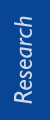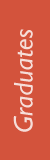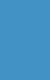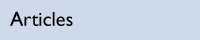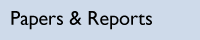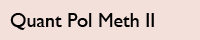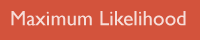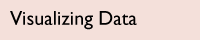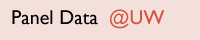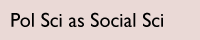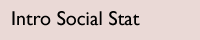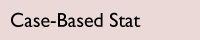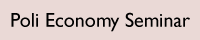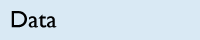Maximum Likelihood Methodsfor the Social Sciences POLS/CSSS 510 Social science data seldom meet the assumptions of the linear regression model taught in introductory statistics courses. Our data often consist of discrete categorizations or counts of events, and may be correlated across periods or clustered by groups. Students will use maximum likelihood methods to derive models appropriate for their own data, learn to communicate their findings to a broad audience, and gain familiarity with statistical programming in R.

POLS/CSSS 510

Maximum Likelihood Methods
for the Social Sciences

Offered every Fall at the
University of Washington

SyllabusReadingsFall 2019

Class meets:
TTh 4:30—5:50 pm
Electrical & Computer Engineering 125

 TA: TBA

Section meets:
F 3:30—5:20 pm
Smith 211

Lectures           PDFs of slides are best viewed in Adobe Acrobat, rather than in your browser.

Topic 1

You may want to read through Kevin Quinn’s matrix algebra and probability distribution reviews, or consult my undergrad lectures on discrete and continuous distributions. For a more general review, you can find the lecture notes for the CSSS Math Camp here.

There are also R code and data for exploratory data analysis using histograms and boxplots, code and data for a simple bivariate linear regression, and code and data for a multiple regression example.

Finally, you’ll find detailed instructions for downloading, installing, and learning my recommended software for quantitative social science here. Focus on steps 1.1 and 1.3 for now, and then, optionally, step 1.2.

Topic 2

The R code to simulate heteroskedastic data and model that data using a heteroskedastic normal maximum likelihood is here.

There are additional mini-lectures on two topics. The first is a review of Bayes Rule. The second presents the Monty Hall problem in color and printable pdf formats. Three versions of the Monte Hall simulation code are available: the first uses a loop and many small steps, the second uses a loop and more compact code, and the third uses lapply() to avoid looping.

Topic 3

There are separate R scripts for interpreting and selecting binary logit models, as well as an example dataset. The goodness of fit code also relies on R functions for computing the percent correctly predicted and making predicted-versus-actual plots and ROC plots, which you should place in your working directory. An example trio of plots showing actual versus predicted probabilities, error versus predicted probabilities, and the ROC curve can be seen here.

Topic 4

R code and data for an ordered probit, which produces graphics for expected value plots and first difference plots.

Topic 5

R code for a multinomial logit, which produces a variety of graphical summaries of a multinomial logit model: for expected values plotted together, expected values plotted separately in a tiled format, first difference plotted for a single scenario and all categories, relative risks plotted for a single scenario and all categories, and relative risks plotted for many scenarios at once.

Topic 6

Two code examples are discussed in this lecture.

The first example analyzes bounded counts using Binomial, Beta-Binomial, and Quasibinomial models of turnout in the 2004 general election in Washington state. Example output includes this plot of expected counts under different models for various counterfactual scenarios. Note this example uses multiple imputation to fill in missing data. You will need:

• The main R script to run the models, cross-validation, and graphics
• Data (csv format) from the Washington Secretary of State & US Census
• An R helper file with cross-validation functions

The second example analyzes unbounded counts using Poisson, Negative Binomial, Quasipoisson, Zero-inflated Poisson, and Zero-inflated Negative Binomial models of foreclosure filings by Houston, Texas area Home Owner Associations (HOAs). Example output includes this plot of expected values from a zero-inflated negative binomial model. You will need:

See the Topic 6 example on turnout for an R code using multiple imputation of missing data. Also available is an example (R script, data, plot) showing the use of overimputation to compute coverage of multiple imputation prediction intervals for real data.

For the curious, the R script used to construct the example plots in the first half of this lecture is here.

Self-Study Lecture 1

This lecture and the two below it introduce log-linear models of tabular data, and will not be presented as part of POLS/CSSS 510. They are posted here for interested students, especially for the use of mosaic plots to investigate cross-tabulated data (in this lecture, and in the third lecture on multidimensional tables). Students interested in a CSSS course on log-linear models should investigate CSSS 536.

Self-Study Lecture 2

Self-Study Lecture 3

Student Assignments

Problem Set 1Due in class Thursday 10 October 2019

Problem Set 2Due in class Tuesday 22 October 2019

Problem Set 3Due in class Tuesday 5 November 2019

Data for problem 1 in comma-separated variable format.

Problem Set 4Due in class Thursday 14 November 2019

Data for problem 1 in comma-separated variable format.

Problem Set 5Due in class Tuesday 26 November 2019

Data for problem 1 in comma-separated variable format; data for problem 2 in R data format.

Problem Set 6Due in class Thursday 5 December 2019

Data will be provided.

Poster Presentations

3 December 2019 to 5 December 2019

Requirements and suggestions for poster presentations will be presented in class.

Final Paper

Due Tuesday 10 December 2019, 3:00 pm, both in my Gowen mailbox and by email

See the syllabus for paper requirements, and see my guidelines and recommendations for quantitative research papers.

Labs

Lab 1

Logistics and R ReviewSupplementary material: R code and data for lab: lab1_code.Rmd, lab1_data.csv and lab1_survey.csv.

Lab 2

Distributions and R PracticeSupplementary material: Complete code for lab: lab2_code.Rmd.

Lab 3

OLS and MLE for heteroskedastic normalSupplementary material: See Chris's R code for simulating and modelling heteroskedastic data under Topic 2; code in slides is based on personal adaptation

Lab 4

OLS and MLE for heteroskedastic normal (cont.)Supplementary material: Starting code for lab: lab4_start_code.R. See Chris's R code for simulating and modelling heteroskedastic data under Topic 2; code in slides is based on personal adaptation

Lab 5

Estimating and Interpreting Logit ModelsSupplementary material: Starting code for lab: lab5_start_code.Rmd; code to visualize expected values from logit models in ggplot2: nesLogit_ggplot2.R.Designed byChris Adolph & Erika SteiskalCopyright 2011–2020Privacy · Terms of Use